NCERT Solutions for Class 8 Maths Chapter 5 Data Handling Ex 5.2 are part of NCERT Solutions for Class 8 Maths. Here we have given NCERT Solutions for Class 8 Maths Chapter 5 Data Handling Ex 5.2.

 Board CBSE Textbook NCERT Class Class 8 Subject Maths Chapter Chapter 5 Chapter Name Data Handling Exercise Ex 5.2 Number of Questions Solved 5 Category NCERT Solutions

## NCERT Solutions for Class 8 Maths Chapter 5 Data Handling Ex 5.2

Question 1.
A survey was made to find the type of music that a certain group of young people liked in a city. The adjoining pie chart shows the findings of this survey.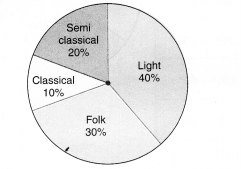From this pie chart answer the following:
(i) If 20 people liked classical music, how many young people were surveyed?
(ii) Which type of music is liked by the maximum number of people?
(iii) If a cassette company were to make 1000 CD’s, how many of each type would they make?
Solution.
(i) Suppose that x young people were surveyed. Then, the number of young people who liked classical music = 10% of x
$$\frac { 10 }{ 100 } \times x=\frac { x }{ 10 }$$According to the question,
$$\frac { x }{ 10 } =20$$
⇒ x = 20 x 10
⇒ x = 200
Hence, 200 young people were surveyed.

(ii) Light music is liked by the maximum number of people.

(iii) Total number of CD’s = 1000 Number of CD’s of Semi Classical music = 20% of 1000
⇒ $$\frac { 20 }{ 100 } \times 1000=200$$
Number of CD’s of Classical music = 10% of 1000
⇒ $$\frac { 10 }{ 100 } \times 1000=100$$
Number of CD’s of Folk music = 30% of 1000
⇒ $$\frac { 30 }{ 100 } \times 1000=300$$
Number of CD’s of Light music = 40% of 1000
⇒ $$\frac { 40 }{ 100 } \times 1000=400$$

Question 2.
A group of 360 people was asked to vote for their favorite season from the three seasons rainy, winter and summer.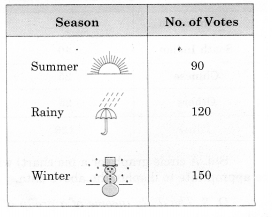(i) Which season got the most votes?
(ii) Find the central angle of each sector.
(iii) Draw a pie chart to show this information.
Solution.
(i) Winter season got the most votes.

(ii) Total votes = 90 + 120 + 150 = 360. Central angle of winter sector
$$= \frac { Number\quad of\quad people\quad who\quad vote\quad for\quad winter\quad season }{ Total\quad number\quad of\quad people } \times { 360 }^{ \circ }$$
= $$\frac { 150 }{ 360 } \times { 360 }^{ \circ }={ 150 }^{ \circ }$$
Central angle of summer sector
$$= \frac { Number\quad of\quad people\quad who\quad vote\quad for\quad summer\quad season }{ Total\quad number\quad of\quad people } \times { 360 }^{ \circ }$$
= $$\frac { 90 }{ 360 } \times { 360 }^{ \circ }={ 90 }^{ \circ }$$
Central angle of rainy sector
$$= \frac { Number\quad of\quad people\quad who\quad vote\quad for\quad rainy\quad season }{ Total\quad number\quad of\quad people } \times { 360 }^{ \circ }$$
= $$\frac { 120}{ 360 } \times { 360 }^{ \circ }={ 120 }^{ \circ }$$

(iii)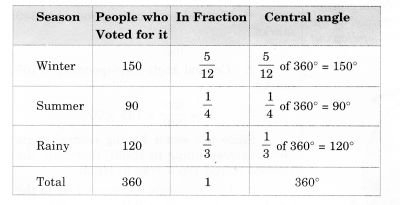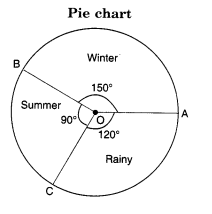Question 3.
Draw a pie chart showing the following information. The table shows the colors preferred by a group of people.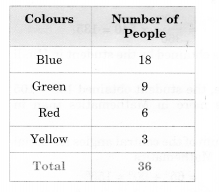Find the proportion of each sector. For example, Blue is $$\frac { 18 }{ 36 } =\frac { 1 }{ 2 }$$ ; Green is $$\frac { 9 }{ 36 } =\frac { 1 }{ 4 }$$ ; and so on. Use this to find the corresponding angles.
Solution.Question 4.
The adjoining pie chart gives the marks scored in an examination by a student in Hindi, English, Mathematics, Social Science and Science. If the total marks obtained by the students were 540, answer the following questions.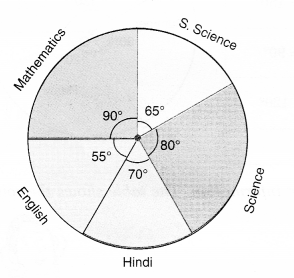(i) In which subject did the student score 105 marks?
(Hint: For 540 marks, the central angle = 360°. So, for 105 marks, what is the central angle ?)
(ii) How many more marks were obtained by the student in Mathematics than in Hindi?
(iii) Examine whether the sum of the marks obtained in Social Science and Mathematics is more than that in Science and Hindi.
(Hint; Just study the central angles).
Solution.
(i) Total marks = 540
∵ Central angle corresponding to 540 = 360°
∴ Central angle corresponding to 105
$$\frac { { 360 }^{ \circ } }{ 540 } \times \left( 105 \right) ={ 70 }^{ \circ }$$
Since the sector having central angle 70° is corresponding to Hindi, therefore, the student scored 105 marks in Hindi.

(ii) Central angle corresponding to the sector of Mathematics = 90°
∴ Marks obtained by the student in Mathematics
$$\frac { { 90 }^{ \circ } }{ { 360 }^{ \circ } } \times 540=135$$.
Marks obtained by the student in Hindi = 105.
Hence, the student obtained 135 – 105 = 30 marks more in Mathematics than in Hindi.

(iii) Sum of the central angles for Social Science and Mathematics
= 65° + 90° = 155°
Sum of the central angles for Science and Hindi
= 80° + 70° = 150°
Since the marks obtained are proportional to the central angles corresponding to various subjects and 155° > 150°, therefore the sum of the marks obtained in Social Science and Mathematics is more than that in Science and Hindi.

Question 5.
The number of students in a hostel, speaking different languages is given below. Display the data in a pie chart.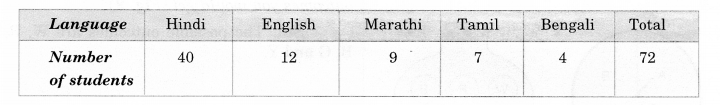Solution.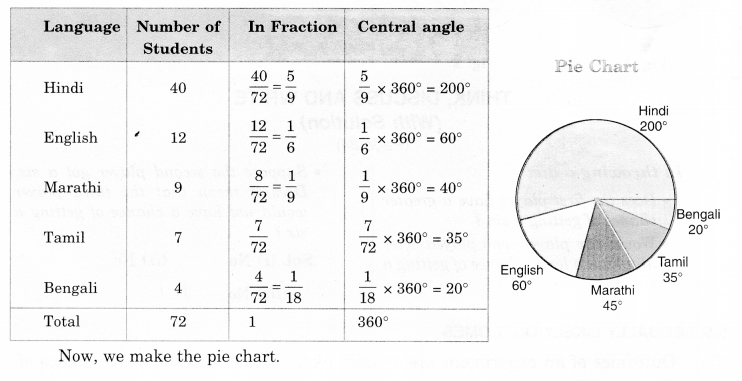We hope the NCERT Solutions for Class 8 Maths Chapter 5 Data Handling Ex 5.2 help you. If you have any query regarding NCERT Solutions for Class 8 Maths Chapter 5 Data Handling Ex 5.2, drop a comment below and we will get back to you at the earliest.